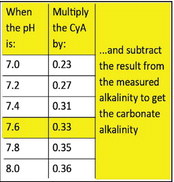Posted on

# extremely corrosive environment. This circumstance ….extremely corrosive environment. This circumstance would cause etching to plaster surfaces as well as corroded metals.

In the case of borate use, its effects become more impactful at higher pH values.

CYA correction

At normal pool pH levels, to calculate carbonate alkalinity, the rule of thumb is to subtract out one-third of the cyanuric acid concentration. In other words, total alkalinity is corrected for cyanurate alkalinity by the following equation to yield carbonate alkalinity:

Alkalinity corrected = Alkalinity total – 1/3 Cyanuric Acid

Thus, if the test for total alkalinity shows 100 ppm, while the test for cyanuric acid shows 90, the carbonate alkalinity is roughly 70.

100 – (.33 x 90) = 70

The one-third correction factor is pH dependent. That is because the cyanuric acid and cyanurate ion concentrations are pH dependent. Therefore, it is best to use correction factors that account for this pH dependency.

The cyanuric acid correction factors may be found in the accompanying table.

The borate correction factor is pH dependent. The borate correction factors may be found in the accompanying table. To obtain the carbonate alkalinity from the measured total alkalinity in the presence of borates, the following procedure can be used.

1. Select a borate factor based on the measured pH: F( B)

2. Calculate the carbonate alkalinity from the measured total alkalinity, the measured borate concentration (B), and the selected borate factor (F(B))

Alkalinity corrected = Alkalinity total – (B x F( B))

For example, if the measured total alkalinity is 100 ppm, the pH is 7.6, and the measured borate concentration is 50 ppm, then:

Alkalinity corrected = 100 – (50 x 0.17) Alkalinity corrected = 92 ppm

SI = pH + F( T) + F( TA) + F( CH)

– F( TDS)

However, a calculated carbonate alkalinity must be used prior to selecting F( TA).

For example, consider the following measured water parameters: pH = 7.6; Temperature = 76; Total Alkalinity = 100 ppm; Cyanuric Acid = 90 ppm; Borates = 50 ppm; Calcium Hardness = 200 ppm; Total Dissolved Solids = 800 ppm First, calculate the carbonate alkalinity, or Alkalinity corrected: The Alkalinity corrected is obtained using the cyanuric acid and borate correction factors and the following formula:

Alkalinity corrected = TA – (CYA X .33) – (B x F( B))

Alkalinity corrected = 100 – (100 x .33) – (50 x 0.17) Alkalinity corrected = 58.5 ppm

Next, find the factors for the Langelier Saturation Index from the accompanying table and simply plug them into the general formula:

LSI =

pH + F( T) + F( TA) + F( CH) – F( TDS) LSI = 7.6 + 0.6 + 1.8 + 1.9- 12.1 LSI = -0.2

This number is a little low, but it is still within range.

Table 2: Cyanuric Acid Correction factors as a function of pH

Borate Correction

Putting it all together

As will soon become apparent, calculating the LSI in the presence of cyanuric acid and borates is a bit complicated, which is why many of today’s pool service techs simply install smart phone LSI calculators to save time.

However, for those interested in learning how to calculate the saturation index by hand, the following formula is applied to the water balance parameters:

Table 3 Borate Correction Factors as a function of pHLATEST NEWS